## Friday, July 29, 2011

### 174

174 = 2 x 3 x 29.

174 is a divisor of 592 - 1.

174 is the number of twin primes among the first thousand primes.

174 = 72 + 53.

174 is the smallest number that can be written as the sum of four positive distinct squares in six ways.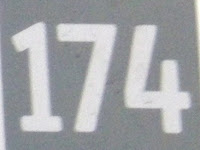In 1978, Landon Noll and Laura Nickel used a CDC Cyber 174 to prove that the Mersenne number M21701 is a prime number.

## Thursday, July 28, 2011

### 709

709 is a prime number.

709 has a representation as a sum of two squares: 709 = 152 + 222.

709 is the hypotenuse of a primitive Pythagorean triple: 7092 = 2592 + 6602.

709 is the smallest prime of the form 59n + 1.

709 is the smallest prime whose cube is the sum of three prime cubes: 7093 = 1933 + 4613 + 6313.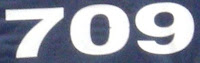709 is the number on General Esperanza's cargo plane in the movie Die Hard 2: Die Harder.

## Wednesday, July 27, 2011

### 160

160 = 25 x 5.

160 has a representation as a sum of two squares: 160 = 42 + 122.

160 is the smallest number that is the sum of three distinct prime cubes: 160 = 23 + 33 + 53.

160! - 159! + 158! - . . . -3! + 2! -1! is a prime number.

Each internal angle of a regular 18-sided polygon (octadecagon) is 160 degrees.160 is the number of square rods in an acre.

## Tuesday, July 26, 2011

### 987

987 = 3 x 7 x 47.

987 is the 16th Fibonacci number.

987 is a divisor of 466 - 1.

987 is the only three-digit number with consecutively decreasing digits such that when multiplied by the sum of its digits plus and minus 1, the results are primes: 987(9 + 8 + 7) - 1 = 23,687 and 987(9 + 8 + 7) + 1 = 23,689.

1 x 8 + 1 = 9
12 x 8 + 2 = 98
123 x 8 + 3 = 987
1234 x 8 + 4 = 9876
12345 x 8 + 5 = 98765987 Hotels has hotels in Prague and Barcelona.

## Monday, July 25, 2011

### 1270

1270 = 2 x 5 x 127.

1270 is a divisor of 196 - 1.

1270 is 1661 in base 9.

1270 is the sum of five positive fifth powers: 1270 = 45 + 35 + 15 + 15 + 15.1270 is a perfume produced by Frapin and named for the year in which the Frapin family established itself in the Cognac region of France.

## Friday, July 22, 2011

### 447

447 = 3 x 149.

447 is a divisor of 444 - 1.

447 is the smallest number of convex quadrilaterals formed by 15 points in general position.

447 is 677 in base 8 and 377 in base 11. It is 313 in base 12.

447 is a number that cannot be written as a sum of three squares.Air France Flight 447 from Rio de Janeiro to Paris crashed into the Atlantic Ocean on June 1, 2009.

## Thursday, July 21, 2011

### 582

582 = 2 x 3 x 97.

582 is the number of antisymmetric relations on a five-element set.

582 is a divisor of 613 - 1.

582 is 210120 in base 3 and 21012 in base 4.

582 is the sum of eight consecutive primes: 582 = 59 + 61 + 67 + 71 + 73 + 79 + 83 + 89.The Rotax 582 is an engine designed for ultralight aircraft.

## Wednesday, July 20, 2011

### 276

276 = 22 x 3 x 23.

276 is the 23rd triangular number.

276 is the magic sum of a magic square made up of 16 consecutive primes.

276 is the largest difference between two consecutive primes less than 109.

276 = 15 + 25 + 35.Loctite 276 is an adhesive for the permanent locking and sealing of threaded fasteners.

## Tuesday, July 19, 2011

### 910

910 = 2 x 5 x 7 x 13.

910 is a divisor of 813 - 1.

910 is 1020201 in base 3, 32032 in base 4, 12120 in base 5, 4114 in base 6, 1616 in base 8, and 1221 in base 9.

910 is a number with 16 divisors: 1, 2, 5, 7, 10, 13, 14, 26, 35, 65, 70, 91, 130, 182, 455, 910.

910 x 913 = 830830.Telephone area code 910 serves the southeastern portion of the state of North Carolina.

## Monday, July 18, 2011

### 294

294 = 2 x 3 x 72.

294 is a divisor of 972 - 1.

2*294 + 9*294 + 4*294 - 1 is a prime number (4409).

294 is the number of planar 2-connected graphs with seven vertices.

144,648 = 861 x 168 = 492 x 294.The Toshiba 8TM-294 was a portable transistor radio produced in 1959 and 1960.

## Friday, July 15, 2011

### 835

835 = 5 x 167.

835 is the ninth Motzkin number.

835 is 343 in base 16.

835 is the sum of six positive cubes in exactly three ways.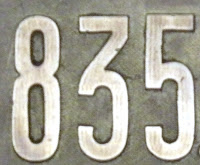Inn at 835 is a historic hotel in Springfield, Illinois.

## Thursday, July 14, 2011

### 267

267 = 3 x 89.

267 is a divisor of 882 - 1.

267 is the smallest number n such that n plus a googol is prime.

267 is the number of planar partitions of 12.

267 is 151 in base 14.

267 is the number of groups of order 64.267 is the telephone area code for parts of eastern Pennsylvania.

## Wednesday, July 13, 2011

### 640

640 = 27 x 5.

640 has a representation as a sum of two squares: 640 = 82 + 242.

100n2 - 640n + 2(640) - 100 + 7 gives distinct primes for n = 0 to 24.

640 is 22000 in base 4 and 1200 in base 8. It is 454 in base 12.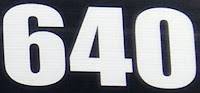640 is the number of acres in a square mile.

## Tuesday, July 12, 2011

### 944

944 = 24 x 59. The sum of its factors is a prime: 2 + 2 + 2 + 2 + 59 = 67.

944 is a divisor of 1729 - 1.

944 starts off a record-breaking run of consecutive integers with exactly five prime factors (counted with multiplicity).

Adding 944 to the sum of its digits produces a square: 944 + 9 + 4 + 4 = 961 = 312.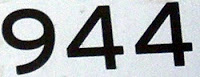The Porsche 944 is a sports car manufactured from 1982 to 1991.

## Monday, July 11, 2011

### 1113

1113 = 3 x 7 x 53.

1113 is the number of integer partitions of 40 into distinct parts.

1113 is divisible by the product of its digits (1 x 1 x 1 x 3 = 3).

1113 is the concatenation of two consecutive primes (11 and 13).

The sum of the divisors of 1113 is a cube: 1 + 3 + 7 + 21 + 53 + 159 + 371 + 1113 = 1728 = 123.1113 Katja is an asteroid discovered in 1928.

## Friday, July 8, 2011

### 279

279 = 32 x 31.

279 can be expressed as a prime raised to three consecutive primes: 279 = 32 + 33 + 35.

279 = (27 x 9) + (27 + 9).

279 is the maximum number of 8th powers needed to sum to any number.

279 is a number that cannot be written as a sum of three squares.The longer side of a standard "letter-size" sheet of paper in the United States is 11 inches or about 279 millimeters.

## Thursday, July 7, 2011

### 923

923 = 13 x 71.

923 multiplied by its successor gives a number concatenated with itself: 923 x 924 = 852,852.

9233 = 786,330,467 = (786 - 330 + 467)3.

923 is 32123 in base 4.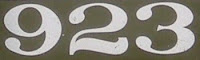Caboose 923 of the Richmond, Fredericksburg & Potomac Railroad was restored by the Chesapeake Railway Association.

## Wednesday, July 6, 2011

### 1036

1036 = 22 x 7 x 37.

1036 is 4444 in base 6.

1036 is a divisor of 434 - 1.

1036 is the maximum number of regions into which 45 lines divide the plane.

1036 is the smallest number whose cube starts with four identical digits: 10363 = 1111934656.1036 Ganymed is the largest Amor asteroid.

## Tuesday, July 5, 2011

### 266

266 = 2 x 7 x 19.

266 is 222 in base 11.

266 is a divisor of 113 - 1.

The palindromic concatenation reflection of 266 (662266) has a prime factorization with only palindromic factors: 662266 = 2 x 11 x 30103.

2,661,653 = 16532 - 2662.The gestation period for humans is about 266 days.

## Friday, July 1, 2011

### 511

511 = 7 x 73.

511 is 111111111 in base 2 (binary). It is 777 in base 8.

511 is a Mersenne number.

511 is the smallest composite number that is the exact average of three consecutive primes (503, 509, and 521): (503 + 509 + 521)/3 = 511.

511 is a number that cannot be written as a sum of three squares.The telephone number 511 is often used for traffic and travel information.